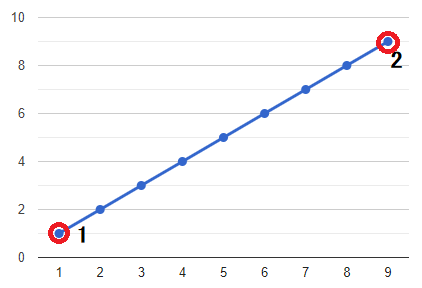# 376. Wiggle Subsequence / Medium

A wiggle sequence is a sequence where the differences between successive numbers strictly alternate between positive and negative. The first difference (if one exists) may be either positive or negative. A sequence with one element and a sequence with two non-equal elements are trivially wiggle sequences.

• For example, `[1, 7, 4, 9, 2, 5]` is a wiggle sequence because the differences `(6, -3, 5, -7, 3)` alternate between positive and negative.
• In contrast, `[1, 4, 7, 2, 5]` and `[1, 7, 4, 5, 5]` are not wiggle sequences. The first is not because its first two differences are positive, and the second is not because its last difference is zero.
A subsequence is obtained by deleting some elements (possibly zero) from the original sequence, leaving the remaining elements in their original order.

Given an integer array nums, return the length of the longest wiggle subsequence of `nums`.

## Example 1:

Input: nums = [1,7,4,9,2,5]
Output: 6
Explanation: The entire sequence is a wiggle sequence with differences (6, -3, 5, -7, 3).

## Example 2:

Input: nums = [1,17,5,10,13,15,10,5,16,8]
Output: 7
Explanation: There are several subsequences that achieve this length.
One is [1, 17, 10, 13, 10, 16, 8] with differences (16, -7, 3, -3, 6, -8).

## Example 3:

Input: nums = [1,2,3,4,5,6,7,8,9]
Output: 2

## Constraints:

• 1 <= nums.length <= 1000
• 0 <= nums[i] <= 1000

# Solution 1: Simply DP

## 效能

### Complexity

• Time Complexity: O(N)
• Space Complexity: O(N)

### LeetCode Result

• Runtime: 5 ms
• Memory Usage: 7.3 MB

# Solution 2: DP with Space O(1)

## 效能

### Complexity

• Time Complexity: O(N)
• Space Complexity: O(1)

### LeetCode Result

• Runtime: 3 ms
• Memory Usage: 7 MB

# Solution 3: Greedy

## 思路

• Example 1 => nums = `[1, 2, 3, 4, 5, 6, 7, 8, 9 ]`• Example 2 => nums = `[1, 17, 5, 10, 13, 15, 10, 5, 16, 8 ]`## 效能

### Complexity

• Time Complexity: O(N)
• Space Complexity: O(1)

### LeetCode Result

• Runtime: 0 ms
• Memory Usage: 7 MB